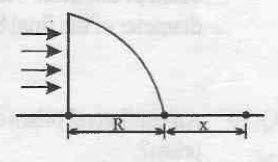# Mystic DarknessA uniform, horizontal beam of light is incident upon a quarter cylinder of radius $\displaystyle R$, and has a refractive index $\displaystyle\mu$. Starting at a distance $x$ from the front of the cylinder, the table is not illuminated.

Find the value of $\displaystyle x$ (in cm).

Details and Assumptions:

• $R = 5cm$
• $\mu = \frac{2}{\sqrt{3}}$
×

Problem Loading...

Note Loading...

Set Loading...